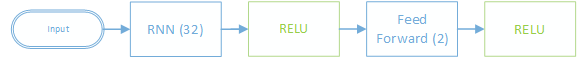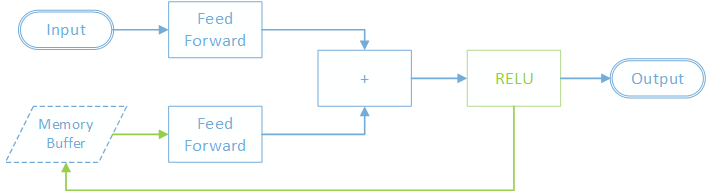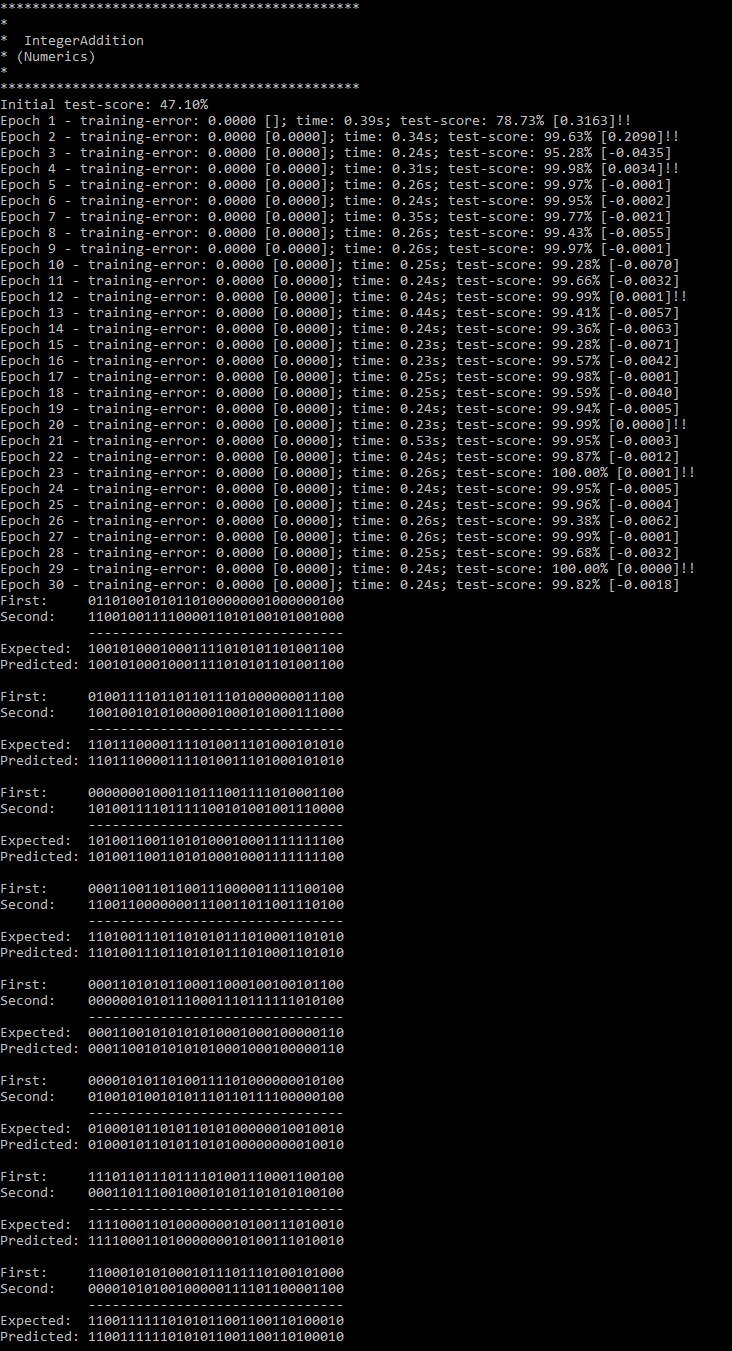# Getting a neural net to learn the rules of binary addition and how to use its memory to store carry bits as appropriate.

## Motivation

Sometimes we want the computer to be able to make predictions based on a sequence of inputs. Each single item in the sequence doesn't contain enough information by itself to make a successful prediction. Instead, the nearby context is needed as well.

One solution is to give a classifier not just the current item but the surrounding items as well. But the problem is that we need to fix the size of the window and the relevant context might sometimes be outside that window.

A better solution is to use recurrent neural networks that use a simple form of memory that allow them to learn from arbitrarily long sequences, and which use their memory to change their predictions based on what they've seen previously in the sequence.

An example of this is binary addition. The rules are simple enough, but the problem is that bits need to "carry" over from previous additions if there is an overflow. The bit might carry across many subsequent additions, so passing a nearby context window isn't always going to work.

Rules

Equals

## Data Format

The sequences that we're going to train on are the bits of each of the two input numbers together with the single output bit at that position of the two numbers added together. For example the training sequence for the above addition is (left to right):

## Generating Data

Bright Wire includes a helper class to generate random sequences of binary integers in the above data format.

We generate 1000 such random integer additions and then split them into training and test sets.

```// generate 1000 random integer additions (split into training and test sets)

## Network DesignFor this example we create a simple recurrent neural network (RNN) with memory size 32 and RELU activation. A second feed forward layer with RELU activation converts the output to size 2.

The graph uses Adam gradient descent optimisation and Gaussian weight initialisation. The error metric is binary classification in which each output is rounded to either 0 or 1.

The initial "memory" for the network is initialised to zero. The network will learn an improved initial memory that is saved into the model when training has completed.

The network is trained using backpropagation through time and with a batch size of 16 and learning rate of 0.01.

```// binary classification rounds each output to either 0 or 1
var errorMetric = graph.ErrorMetric.BinaryClassification;

// configure the network properties
graph.CurrentPropertySet
.Use(graph.GaussianWeightInitialisation(false, 0.3f, GaussianVarianceCalibration.SquareRoot2N))
;

// create the engine
var trainingData = graph.CreateDataSource(Training);
var testData = trainingData.CloneWith(Test);
var engine = graph.CreateTrainingEngine(trainingData, learningRate: 0.01f, batchSize: 16);

// build the network
const int HIDDEN_LAYER_SIZE = 32, TRAINING_ITERATIONS = 30;
graph.Connect(engine)
;

// train the network for twenty iterations, saving the model on each improvement
ExecutionGraphModel? bestGraph = null;
engine.Train(TRAINING_ITERATIONS, testData, errorMetric, bn => bestGraph = bn.Graph);```

## Under the HoodThe SimpleRecurrent layer is composed of several sub nodes that use the supplied memory buffer as follows:

First, the input is fed into a feed forward layer. At the same time, the supplied memory is loaded into a buffer and then fed into another feed forward layer. The output of these two layers are added together and then activated. The output from the activation is then saved back to the buffer.

As the next item in the sequence arrives it is fed into the feed forward layer as above, but instead of loading the initial memory buffer as before, the second feed forward layer gets the output that was saved from the previous time around.

During backpropagation this all happens in reverse, however the supplied memory is only updated when backpropagating (through time) the first item in the sequence.

## Training and Evaluation

The best found graph is saved while training for 30 epochs. Simple recurrent neural networks can be a bit fiddly - they sometimes crash and burn while learning and other times they perform brilliantly. If your network fails to learn, try running it again ; )

After training a graph execution engine is created with the highest scoring set of parameters and executed against 8 unseen integer addition pairs. The output is grouped and written to the console.

```// export the graph and verify it against some unseen integers on the best model
var executionEngine = graph.CreateEngine(bestGraph ?? engine.Graph);
var results = executionEngine.Execute(testData2).ToArray();

// group the output
var groupedResults = new (Vector<float>[] Input, Vector<float>[] Target, Vector<float>[] Output);
for (var i = 0; i < 8; i++) {
var input = new Vector<float>;
var target = new Vector<float>;
var output = new Vector<float>;
for (var j = 0; j < 32; j++) {
input[j] = results[j].Input[i];
target[j] = results[j].Target![i];
output[j] = results[j].Output[i];
}

groupedResults[i] = (input, target, output);
}

// write the results
foreach (var (input, target, output) in groupedResults) {
Console.Write("First:     ");
foreach (var item in input)
WriteAsBinary(item);
Console.WriteLine();

Console.Write("Second:    ");
foreach (var item in input)
WriteAsBinary(item);
Console.WriteLine();
Console.WriteLine("           --------------------------------");

Console.Write("Expected:  ");
foreach (var item in target)
WriteAsBinary(item);
Console.WriteLine();

Console.Write("Predicted: ");
foreach (var item in output)
WriteAsBinary(item);
Console.WriteLine();
Console.WriteLine();
}```

## Output

Final accuracy is around 100%.## Complete Source Code

View the complete source on GitHub